# Definition

• the characteristics of the radios
• the characteristics of the antennas
• the distance between the radios
• the allowed power!

Transmit power: it is expressed in milliwatts or in dBm

• it is always expressed as a negative dBm (-dBm)
• it is the lowest power of signal the radio can distinguish
• the minimum RSL is dependent upon rate, and the lowest rate (1 Mbps) has the greatest sensitivity
• the minimum will be in the range of -75 to -95 dBm
• should be provided by the manifacturer

# Characteristics of the antennas

Antennas are passive devices that create the effect of amplification by virtue of their physical shape

• antennas have the same characteristics when receiving and when transmitting
• a 12 dBi antenna is simply a 12 dBi antenna!
• omnidirectional antennas have a gain of 5-12 dBi
• sectorial antennas have a gain of 12-15 dBi
• parabolic antennas have a gain of 19-24 dBi

# Characteristics of the antennas

Part of the signal's energy is lost in the cables, the connectors and other devices going from the radios to the antennas

• the loss depends on the type of cable used and on its length
• signal loss for short coaxial cables including connectors is quite low, in the range of 2-3 dB
• it is better to have cables as short as possible!

# Path Loss

When calculating the path loss, several effects must be considered: free space loss, attenuation and scattering.

Free space loss: the signal power is diminished by geometric spreading of the wavefront. The further away the two radios, the smaller the received signal. This is independent from the environment, depending only on the distance.

Using decibels to express the loss, and using 2.45 GHz as the signal frequency, the free space loss is:

Lfsl=40 + 20*log(r)

where Lfsl is expressed in dB and r is the distance in meters.

# Path Loss

Attenuation takes place as some of the signal power is absorbed when the wave passes through solid objects as trees, walls, windows, etc.

It is very difficult to quantify. The most convenient way is to express its contribution is by adding an "allowed loss" to the free space loss. For example, experience shows that trees add 10 to 20 dB loss per tree in the direct path.

Taking into account the attenuation, the path loss is then:

Lfsl=40 + 20*log(r) + L(allowed)

where Lfsl is expressed in dB and r is the distance in meters.

# Path Loss

Scattering takes place as some of the RF energy reaches the receiving antenna directly, while some bounces off the ground. Part of the RF energy which bounces off the ground reaches the receiving antenna, but later than the direct signal.

This effect is called multipath, fading or signal distortion.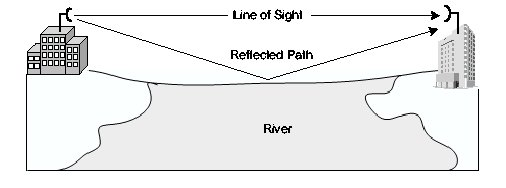# Path Loss

In some cases, the two signals add together, and cause no problem. When the two signals add together out of phase, the received signal is almost worthless: this is known as nulling.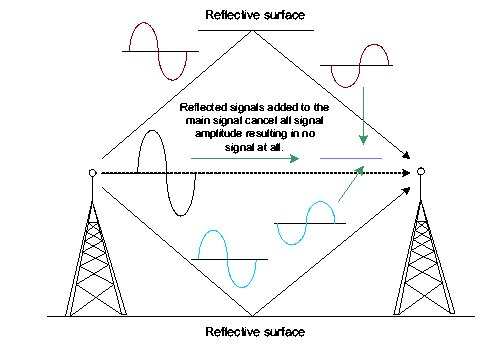# Path Loss

A technique used to deal with multipath is antenna diversity: it consists of adding a second antenna to the radio. Multipath is a very location-specific phenomenon.

Antenna switching diversity: multiple antennas on multiple inputs, with one receiver. In transmission, the last used antenna is used.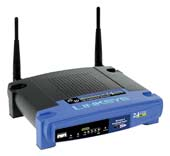# Path Loss

A simple way to take into account scattering in the calculation of the path loss, is to change the exponent of the distance factor of the free space loss formula. The more the scattering, the higher the exponent. For an outdoor environment with tress, an exponent of 3 can be used, while one of 4 for an indoor environment.

Taking into account scattering and attenuation, the path loss is then:

Lfsl=40 + 10*n*log(r) + L(allowed)

where Lfsl is expressed in dB and r is the distance in meters.

# Path Loss

In practice, just the free space loss is calculated. The environment can bring further signal loss, and should be considered for the exact evaluation of the link.

To evaluate if a link is feasible, one must know the characteristics of the equipment being used, and evaluate the path loss.

If you are using two different radios, you should perform the calculation twice, once for each direction!

# Allowed Power

When performing a link budget calculation, we must take into account the power limits which are imposed by the regulatory boards (FCC in the US).

We define an intentional radiator as the RF device and all cabling and connectors up to, but not including, the antenna.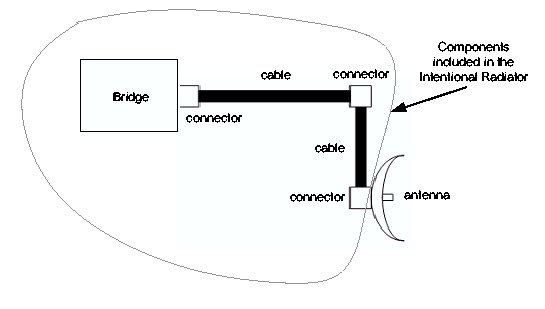# Allowed Power

Any reference to power output of the Intentional Radiator refers to the power output at the end of the last cable or connector before the antenna.

If a 30 mW transmitter loses 15 mW of power in the cables and another 5 mW from the connectors, the power of the intentional radiator is 10 mW (30-15-5=10).

# Allowed Power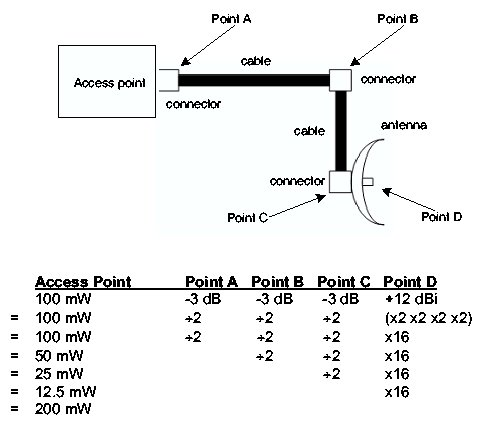# Allowed Power

The EIRP is the Equivalent Isotropically Radiated Power.

EIRP is the power actually radiated by the antenna element and is important because

• it is regulated by the FCC or other regulatory agency
• it is used in calculating whether or not a wireless link is viable

# Allowed Power

The EIRP takes into account the gain of the antenna.

The FCC has rules defining both the power output at the intentional radiator and the antenna element.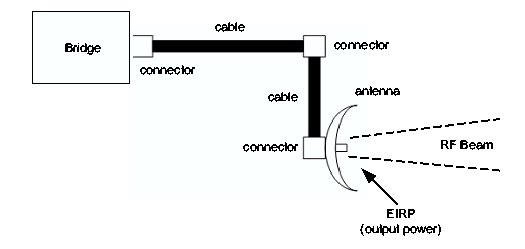# Allowed Power

In the setup of a PtMP link, the FCC limits the EIRP to 4 Watts.

The power limit set for the intentional radiator is 1 Watt.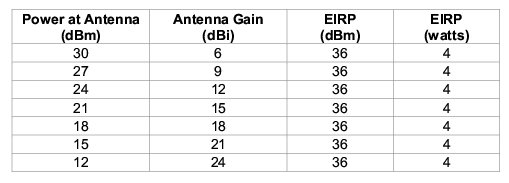# Allowed Power

Ptp links include a single directional transmitting antenna and a single directional receiving antenna.

In the case of PtP links, the power of the intentional radiator is still limited to 1 Watt, but the limit of the EIRP increases with the gain of the antenna

The FCC mandates that for every 3 dBi above the initial 6 dBi of antenna gain, the power at the intentional radiator must be reduced by 1 dB from the initial +30 dBm

# Allowed Power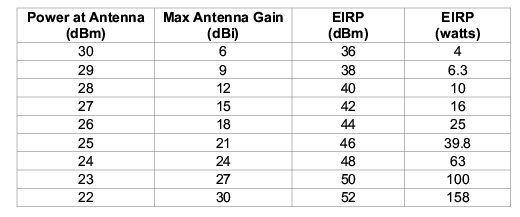If the powers used are within the limits imposed by the regulatory boards, then we can perform the link calculation.

Adding up all the gains and subtracting all the losses gives:

-------------------------
Total Gain

Subtracting the Path Loss from the Total Gain:

Total Gain -
Path Loss
-------------------------
Signal Level at one side of the link

If the resulting signal level is greater than the minimum received signal level, then the link is feasible!

On a given path, the variation of path loss over time can be large, so a certain link margin should be considered. A margin of 10-15 dB is fine. To take into account attenuation and multipath, a margin of 20 dB should be safe enough.

# Example of Link Budget Calculation

We want to estimate the feasibility of a 5 km link, with one AP and one client.

Access Point:

• Tx power= 100mW (20 dBm)
• Sensitivity= -89 dBm
• Antenna= Omni with 10 dBi gain
• Cables are short, with a loss of 2 dB

# Example of Link Budget Calculation

Client:

• Tx power= 30mW (15 dBm)
• Sensitivity= -82 dBm
• Antenna= Sectorial with 14 dBi gain
• Cables are short, with a loss of 2 dB

# Example of Link Budget Calculation

Adding up all the gains and subtracting all the losses gives:

20 dBm (TX Power AP) +
10 dBi (Antenna Gain AP) -
2 dB (Cable Losses AP) +
14 dBi (Antenna Gain Client) -
2 dB (Cable Loses Client)
-------------------------
40 dB (Total Gain)

# Example of Link Budget Calculation

The path loss for a 5 km link, considering only the free space loss, is:

Path Loss= 40 + 20*log(5000) = 113 dB

Subtracting the path loss from the total gain gives:

40 dB - 113 dB = -73 dB

Since -73 is greater than -82 (receive sensitvity of the client), the signal level is enough for the client to be able to hear the AP. There is only 9 dB of margin, which may not be enough to protect against extreme weather conditions.

# Example of Link Budget Calculation

Now we calculate the link from the Client back to the AP:

15 dBm (TX Power Client) +
14 dBi (Antenna Gain Client) -
2 dB (Cable Losses Client) +
10 dBi (Antenna Gain AP) -
2 dB (Cable Loses AP)
-------------------------
35 dB (Total Gain)

# Example of Link Budget Calculation

The path loss is the same on the return trip.

The received signal on the AP is then:

35 dB - 113 dB = -78 dB

Since -78 is greater than -89 (receive sensitvity of the AP), the signal level is enough for the AP to be able to hear the client. There is 11 dB of margin. Using a 24 dBi dish on the client side rather than the 14 dBi antenna, would add an additional 10 dB of gain on both directions of the link.

# Sw for Link Budget Calculation

There are online tools to calculate the link budget.